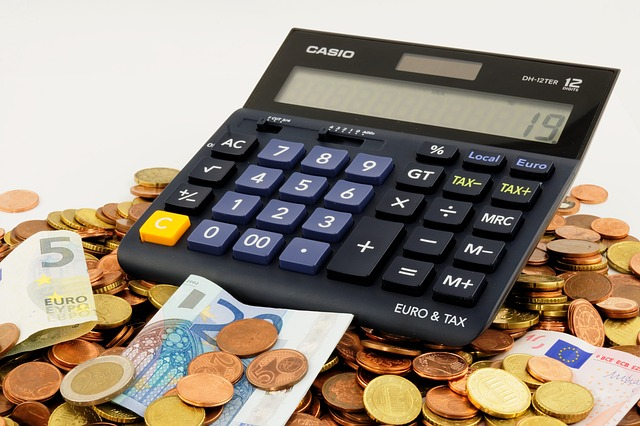Analog computers were first invented in the 1960s. The first calculator was made also in this period with the help of analog computing technology.

With the invention of the microprocessor, the pocket-size calculator first evolved in the 1970s. IC (integrated circuits) made calculators small in size and low cost. So, people started using it for their daily uses.

Calculators are used for many purposes from simple arithmetic operations to complex number calculations in the field of science and finance.

Before purchasing a calculator, you’ve to consider a lot of things. In this article, I will focus on some of the most important factors you must consider before buying a calculator you.

Let’s start.

At first, you’ve to know the types of calculators. From these discussions, you will be able to know which will be best for your purposes.

## Basic Calculator

What will you get in a basic calculator?

• Numeric keyboards with digits
• Simple arithmetical operations (plus, minus, multiplication, division)
• Typically shows 10 digits in its display
• You will not be able to solve fractional problems. Basic calculators convert fractions to decimals and then perform the operations
• In most basic calculators, you will be able to store at least one number in its memory## Scientific Calculators

These are the calculators that made the stage for the graphical calculator. Scientific calculators are widely used to calculate various types of equations in fields like science, engineering, and mathematics. Normally these calculators will have a single line display but this single line is able to show a lot of digits. You will know about graphing calculator after a while. The single most difference between a graphing calculator and a scientific calculator is their display. The Graphing calculator is able to show 2D and 3D charts with colors. Choosing a quality scientific calculator might seem tricky for many students. This guide will help you to select the best suitable one for your purposes.

Here is a lit of calculations that you can do with a scientific calculator:

• Scientific notation of numbers
• Calculate logarithmic values of numbers
• Operations based on exponentially noted numbers
• Finding Trigonometric values of numbers
• Arithmetic based on floating-point numbers
• Operations with complex numbers
• Working with fractional numbers
• Statistical operations
• Equation solving
• Even you can do calculus with a scientific calculator

## Financial Calculators

If you work in a bank or your job role is related to finance, then this calculator is for you.

Including the features of a basic calculator, these calculators can solve these financial and financial problems:

• Present Value (PV) Feature
• Future Value (FV) Calculations
• Amortization (AMORT)
• And a lot of functions related to business, finance, and accounting

## Graphing Calculators

Graphical calculators were first invented by the Casio company. These calculators are designed to show graphs on its screen. You will also be able to solve multiple equations with this calculator. You will also be able to program this calculator in your own way.

These are the areas where graphing calculators are used widely:

• Scientific calculations
• Engineering problems
• And educational purposes

It is easily differentiable a graphical calculator from a general-purpose calculator. Graphical calculators have widescreen because they are designed to show graphs for users. The large screen easily shows charts in the X-Y plane. In today’s world, most graphical calculators are able to show graphs in X-Y-Z (3D) plane and with colors.

Generally, graphing calculators are Wi-Fi capable.

## Printing Calculators

A printing calculator is not different than a basic calculator. The only difference is you can print every input you place in a printing calculator that you cannot do with a basic calculator. Accountants use printing calculators a lot for their works. These users input line by line and they want to keep their inputs as records. Cash registers also use printing calculators but their calculators have some added functionalities for easy tracking of their retail sales.

## Using Calculator as a Teaching Tool

Some instructors like to use a calculator as a teaching tool. Normally they do it in their upper-level classes of mathematics and science. Students cannot easily see a small-sized calculator. So, some manufacturers make specialized calculators for classrooms.

## Final Words

A calculator is a very useful instrument for both study and business purposes. This simple form of the machine can save a lot of time and toil. So, when you will buy one, try to choose one that will fit your purpose well. If your job or work demands a basic calculator, spending a hundred bucks for a graphical calculator is just a huge waste of money and effort.

(Visited 243 times, 1 visits today)